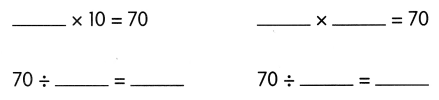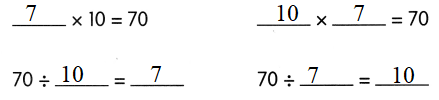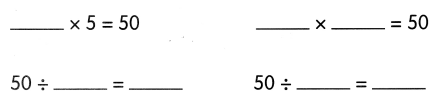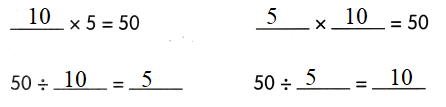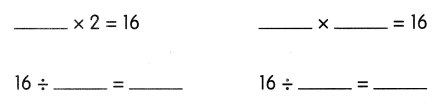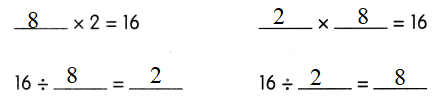# Math in Focus Grade 2 Chapter 6 Practice 6 Answer Key Divide Using Related Multiplication Facts

Go through the Math in Focus Grade 2 Workbook Answer Key Chapter 6 Practice 6 Divide Using Related Multiplication Facts to finish your assignments.

## Math in Focus Grade 2 Chapter 6 Practice 6 Answer Key Divide Using Related Multiplication Facts

Complete the multiplication sentences. Then complete the division sentences.

Example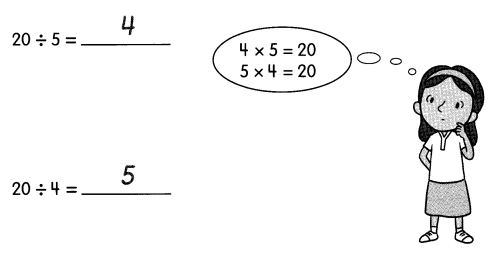Question 1.
4 × ___ = 8
____ × 4 = 8
8 ÷ 2 = ____
8 ÷ 4 = ____
4 × 2 = 8
2 × 4 = 8
8 ÷ 2 = 4
8 ÷ 4 = 2

Question 2.
5 × ___ = 25
____ × 5 = 25
25 ÷ 5 = ___
5 × 5 = 25
5 × 5 = 25
25 ÷ 5 = 5

Question 3.
___ × 2 = 12
2 × ___ = 12
12 ÷ 2 = ___
12 ÷ 6 = ____
6 × 2 = 12
2 × 6 = 12
12 ÷ 2 = 6
12 ÷ 6 = 2

Question 4.
___ × 5 = 15
5 × ___ = 15
15 ÷ 5 = ___
15 ÷ 3 = ____
3 × 5 = 15
5 × 3 = 15
15 ÷ 5 = 3
15 ÷ 3 = 5

Question 5.
___ × 10 = 70
10 × ___ = 70
70 ÷ 10 = ___
70 ÷ 7 = ____
7 × 10 = 70
10 × 7 = 70
70 ÷ 10 = 7
70 ÷ 7 = 10

Question 6.
___ × 2 = 14
2 × ___ = 14
14 ÷ 2 = ___
14 ÷ 7 = ____
7 × 2 = 14
2 × 7 = 14
14 ÷ 2 = 7
14 ÷ 7 = 2

Question 7.
___ × 10 = 60
10 × ___ = 60
60 ÷ 10 = ___
60 ÷ 6 = ____
6 × 10 = 60
10 × 6 = 60
60 ÷ 10 = 6
60 ÷ 6 = 10

Question 8.
___ × 5 = 35
5 × ___ = 35
35 ÷ 5 = ___
35 ÷ 7 = ____
7 × 5 = 35
5 × 7 = 35
35 ÷ 5 = 7
35 ÷ 7 = 5

Use related multiplication facts to solve.

Question 9.
The art teacher divides 30 beads equally among 5 children. How many beads does each child get?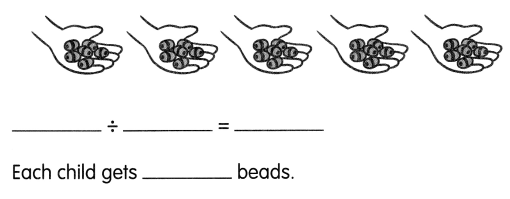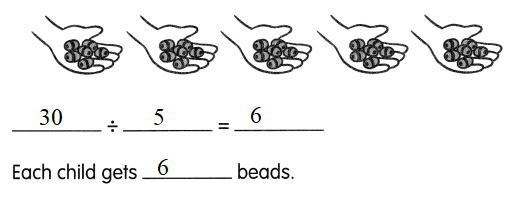Question 10.
Jeff uses 40 craft sticks to make squares of the same size. He makes 10 squares like the one shown. How many craft sticks does he use to make 1 square?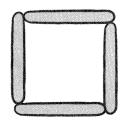_______ ÷ _______ = ______
He uses ____ craft sticks to make 1 square.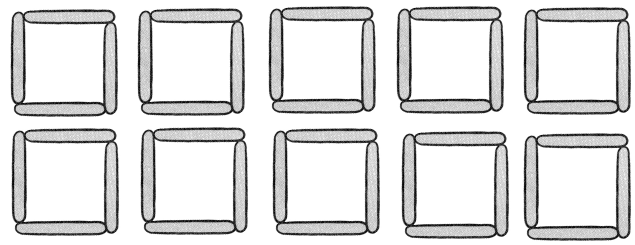40 ÷ 10 = 4
He uses 4 (four) craft sticks to make 1 (one) square.

Question 11.
Mr. Lee has 16 notebooks. He gives each student 2 notebooks. How many students does Mr. Lee have?
_____ ÷ ____ = _____
Mr. Lee has _______ students.
16 ÷ 2 = 8
Mr. Lee has 8 (Eight) students.

Find the missing number.
Then, write one related multiplication sentence. Write two related division sentences.

Example
3 × 2 = 6
6 ÷ 2 = 3

2 × 3 = 6
6 ÷ 3 = 2

Question 12.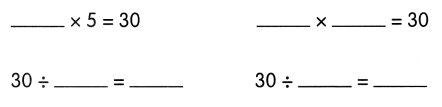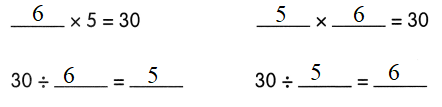Question 13.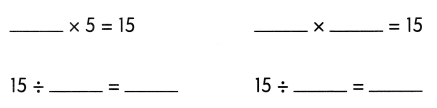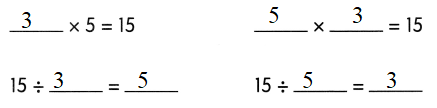Question 14.